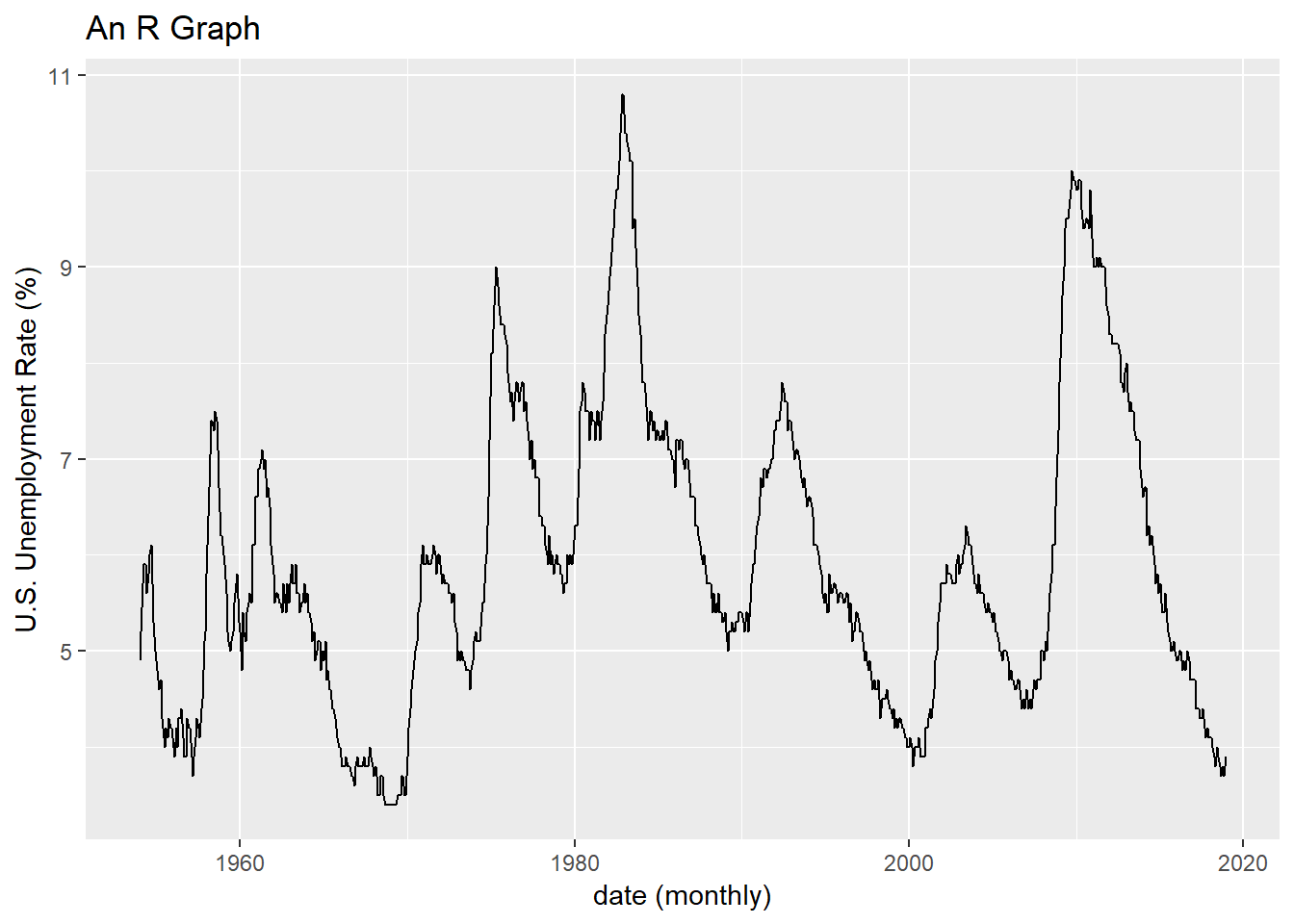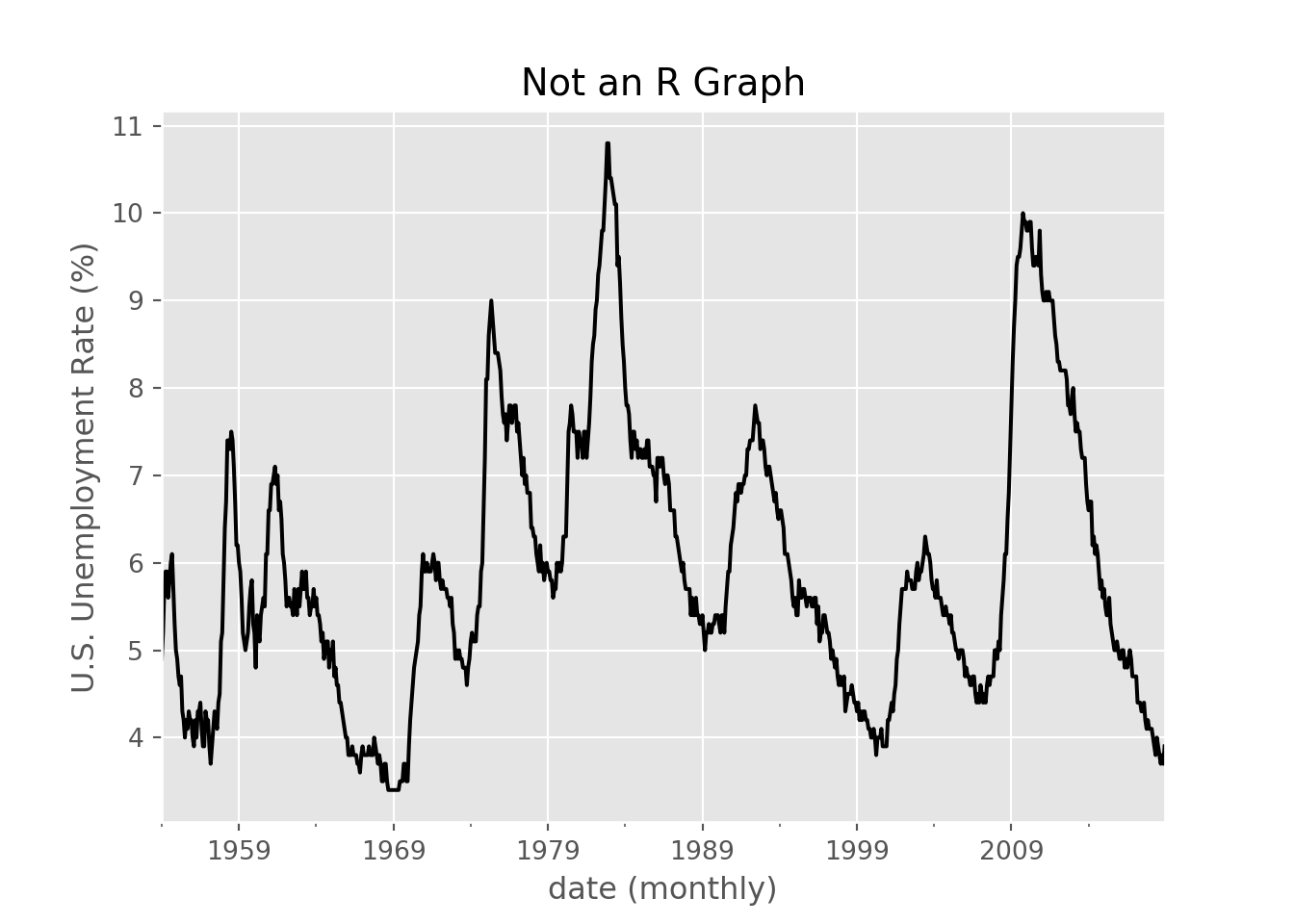# Running Python from R with Reticulate

## Boom

Because reasons I’ve been interested in picking up some Python. But I like the Rstudio IDE, so it sure would be nice if I could just run Python from R. Fortunately, that’s possible using the reticulate package. Let’s give it a try.

Our strategy will be to use R to do the data wrangling and then pass the data to Python to make a plot. Is this a good idea? No, probably not. But it will help to bridge the gap between the familiar (R for me) and the unfamiliar (Python for me).

# Setup

In order for this to work, you’ll need to have a few things, including

• A working version of R
• required packages for plots (tidyverse, tidyquant)
• the reticulate package
• A working version of Python
• required modules for plots (matplotlib, pandas, numpy)
• rpy2 module

Now unfortunately, installation of rpy2 is a little tricky on Windows. For many python packages you can type something like python -m pip install datetime from the cmd line and install the module (datetime in this example), much like you can run install.packages(ggplot2) from R. But for rpy2 this doesn’t work. Windows users could get binaries for rpy2 from an unofficial source maintained by Christoph Gohlke http://www.lfd.uci.edu/~gohlke/pythonlibs/. But the files are stored as .whl files, so tracking through Stackoverflow I found this helpful answer on installing correctly: https://stackoverflow.com/questions/27885397/how-do-i-install-a-python-package-with-a-whl-file.

Once you have that set up, you should be okay to go. I’m sure there are other things you’ll want to do to get started more seriously with Python, but this worked for me today. Which is all right considering where I was this morning:

## Get Data

We’ll keep our data simple and grab the U.S. unemployment rate via FRED as we did in this post.

### Some R code for data wrangling

This code generates a simple data frame with a date variable called date and another column with the U.S. unemployment rate called price (tidyquant syntax).

# get data in R
#####################################################################################
## Step 1: Load Libraries ###
#####################################################################################
library(tidyverse)
library(tidyquant)

#####################################################################################
## Step 2: go get data ###
#####################################################################################

# Set up tickers
tickers<- c("UNRATE")  # unemployment rate from BLS

df<-tq_get(tickers,                         # get selected symbols
get="economic.data",             # use FRED
from="1954-01-01")               # go from 1954 forward

Now print the head of the data with R.

head(df)
## # A tibble: 6 x 2
##   date       price
##   <date>     <dbl>
## 1 1954-01-01   4.9
## 2 1954-02-01   5.2
## 3 1954-03-01   5.7
## 4 1954-04-01   5.9
## 5 1954-05-01   5.9
## 6 1954-06-01   5.6

Now make R run python by using reticulate::use_python to point to Python.

library(reticulate)
use_python("path_to_python",required=TRUE) #set path_to_python to something like C:/Python/Python37/python.exe

Now we can pass the data in dataframe df to Python using r.df. Note that at present, you won’t be able to see the results in Rstudio (running chunks throw an error), but if you compile the document the results show up.

import numpy
print(r.df.head())
##         date  price
## 0 1954-01-01    4.9
## 1 1954-02-01    5.2
## 2 1954-03-01    5.7
## 3 1954-04-01    5.9
## 4 1954-05-01    5.9

# Plot with R

Let’s make a standard R plot

ggplot(data=df, aes(x=date,y=price))+geom_line()+
labs(
y='U.S. Unemployment Rate (%)',
x="date (monthly)",
title="An R Graph")Figure 1: Made with R ggplot2. Data Source: U.S. Bureau of Labor Statistics, Civilian Unemployment Rate [UNRATE], retrieved from FRED, Federal Reserve Bank of St. Louis; https://fred.stlouisfed.org/series/UNRATE, January 28, 2019.

# A Python Plot

Now we can replicate the R plot with Python:

import matplotlib.pyplot as plt
plt.style.use('ggplot')
r.df.plot(x="date",y="price",color="black")
plt.gca().get_legend().remove()
plt.title('Not an R Graph')
plt.xlabel('date (monthly)')
plt.ylabel('U.S. Unemployment Rate (%)')
plt.show()Figure 2: Made with Python matplotlib. Data Source: U.S. Bureau of Labor Statistics, Civilian Unemployment Rate [UNRATE], retrieved from FRED, Federal Reserve Bank of St. Louis; https://fred.stlouisfed.org/series/UNRATE, January 28, 2019.

# More to learn

Where are we going? I’m not sure exactly, but I added a Python tag where we can collect visualizations and analysis we do with Python.

There are some interesting visualizations for Python, for example matplotlib gallery and seaborn gallery. Those might be fun to try out.

Right now it’s baby steps for me as I learn Python as an R user.

I found many helpful suggestions in this thread, thanks to all who responded with some useful links. Check the replies.